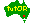# Row Operations

G'day:

We assume that you are familiar with the formal definitions of the basic row operations of linear algebra. If this is not so, you are invited to visit our ROWPS Induction Centre for basic training.

The main objective of this page is to provide you with information on how these operations have been implemented and how you should go about using them. We shall let them introduce themselves shortly, but before we do this it would be useful to display the control panel for these operations and briefly describe how matrices will be displayed on the screen.

For this reason the interface has been deactivated. You are welcome to use it, but do not expect any exciting response. If you want to play with the controls go to where the action is.

In any case, the (deactivated!) control panel is as follows:

 Ri < = > Rj R'i = aRi R'i = Ri + aRj Pivot (i,j)

As you can see, there are four operations and an input field. The later is used to specify a scalar, called a, needed by two of the operations. In this example a=2/3.

It will also be useful to show you how the matrix you'll operate on will appear on the screen and what buttons will be attached to it so that you can communicate with the operations. Here is a sampleThe rules are few and simple:

• If you need to specify only one row use the buttons on the left-hand side of the matrix.
• If you have to specify two rows use the buttons on both sides (what else?!?!) of the matrix.
• If you need to specify a column use the buttons at the bottom of the matrix.
• If you have to specify a (scalar) multiplier, use the input field in the control panel.

There are two counters on the matrix. The one on the left displays the time (in seconds) elapsed since you commenced your session. The one of the right counts how many operations have been conducted.

That's it!

You now have a choice between two options: